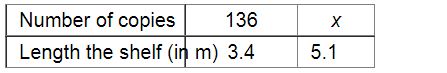# In a library 136 copies of a certain book require a shelf-length of 3.4 metre.`
Question:

In a library 136 copies of a certain book require a shelf-length of 3.4 metre. How many copies of the same book would occupy a shelf-length of 5.1 metres?

Solution:Let $x$ be the number of copies that would occupy a shelf-length of $5.1 \mathrm{~m}$.

Since the number of copies and the length of the shelf are in direct variation, we have:

$\frac{136}{x}=\frac{3.4}{5.1}$

$\Rightarrow 136 \times 5.1=x \times 3.4$

$\Rightarrow x=\frac{136 \times 5.1}{3.4}$

$=204$

Thus, 204 copies will occupy a shelf of length $5.1 \mathrm{~m}$.